# Quantum Numbers Activity Quantum Numbers Quantum numbers tell

• Slides: 24Quantum Numbers Activity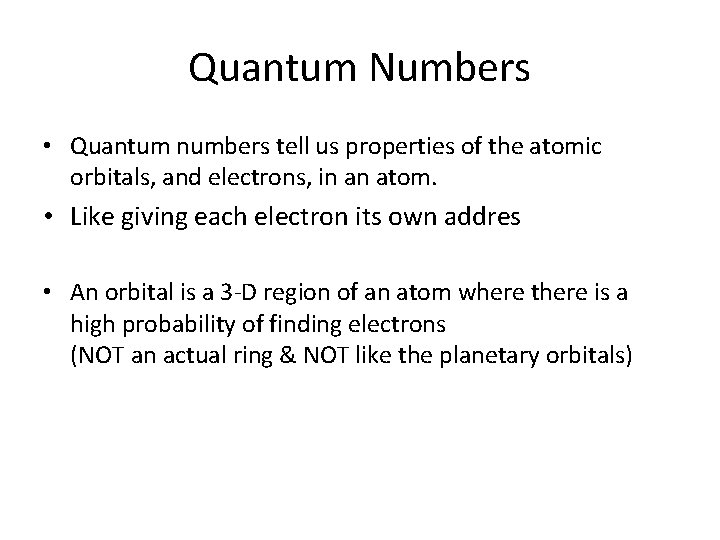Quantum Numbers • Quantum numbers tell us properties of the atomic orbitals, and electrons, in an atom. • Like giving each electron its own addres • An orbital is a 3 -D region of an atom where there is a high probability of finding electrons (NOT an actual ring & NOT like the planetary orbitals)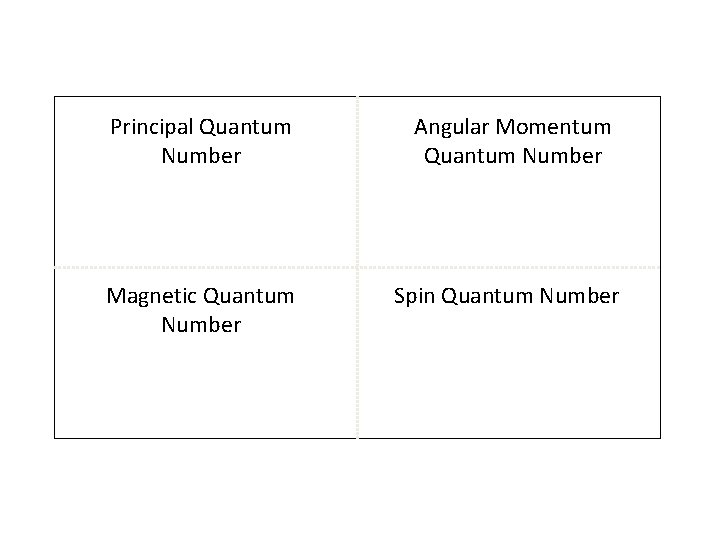Principal Quantum Number Angular Momentum Quantum Number Magnetic Quantum Number Spin Quantum NumberTextbook • You will need a textbook to look up information about the quantum numbers. Pg. 101.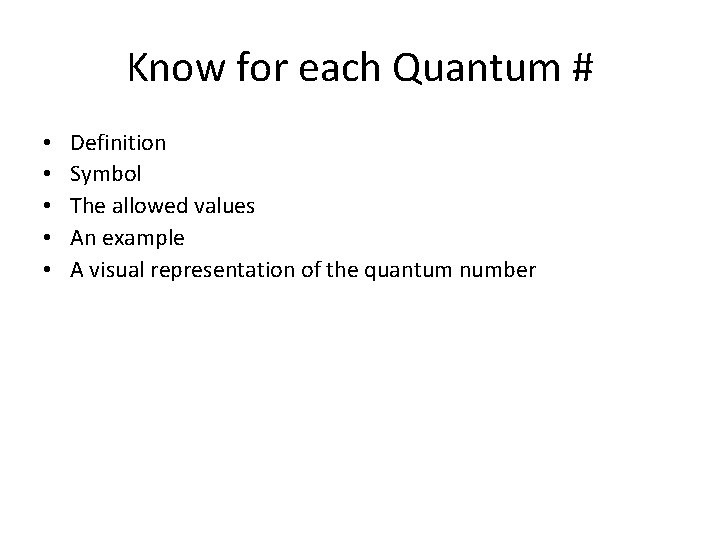Know for each Quantum # • • • Definition Symbol The allowed values An example A visual representation of the quantum number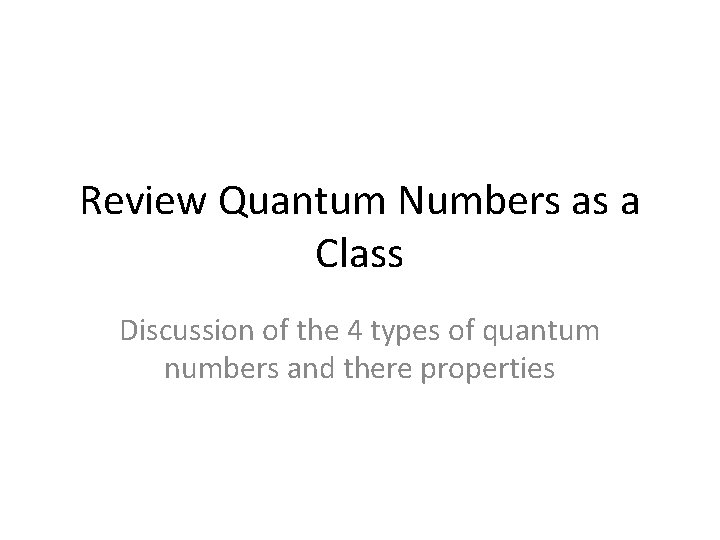Review Quantum Numbers as a Class Discussion of the 4 types of quantum numbers and there properties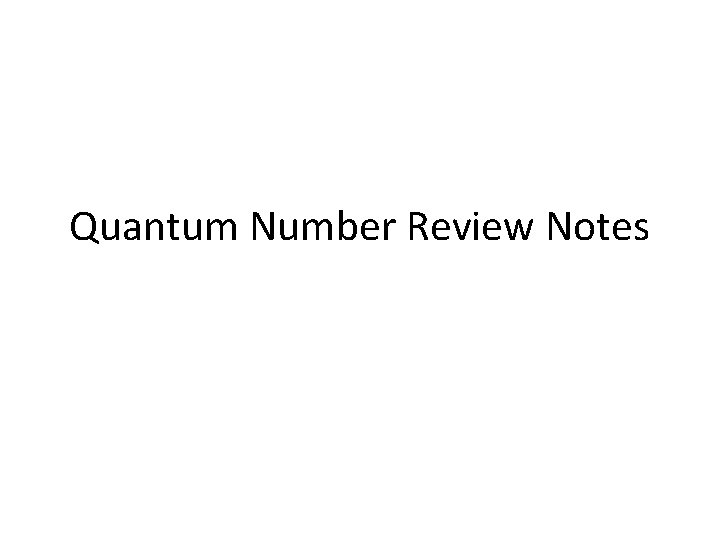Quantum Number Review Notes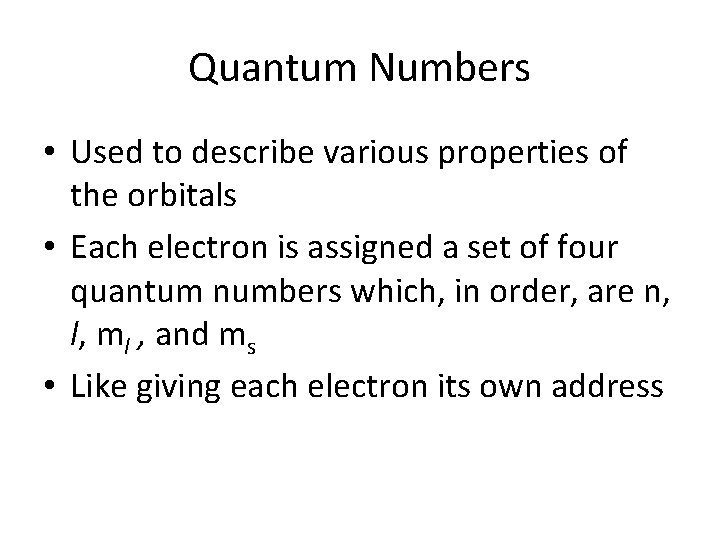Quantum Numbers • Used to describe various properties of the orbitals • Each electron is assigned a set of four quantum numbers which, in order, are n, l, ml , and ms • Like giving each electron its own address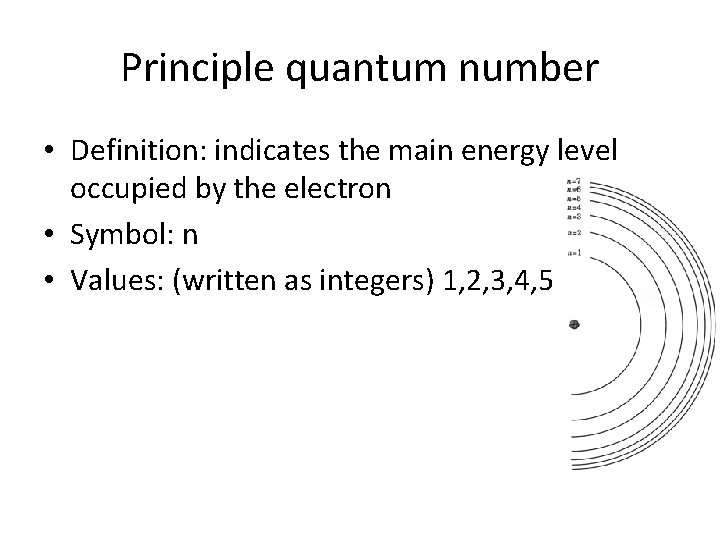Principle quantum number • Definition: indicates the main energy level occupied by the electron • Symbol: n • Values: (written as integers) 1, 2, 3, 4, 5, 6, 7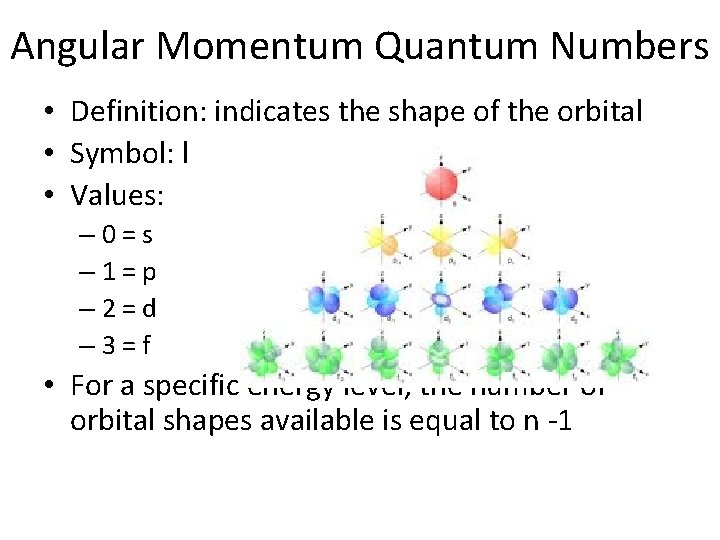Angular Momentum Quantum Numbers • Definition: indicates the shape of the orbital • Symbol: l • Values: – 0=s – 1=p – 2=d – 3=f • For a specific energy level, the number of orbital shapes available is equal to n -1Magnetic Quantum Numbers • Definition: represents the orientation of an orbital around the nucleus • Symbol: ml • Values: for a p-orbital -1, 0 , 1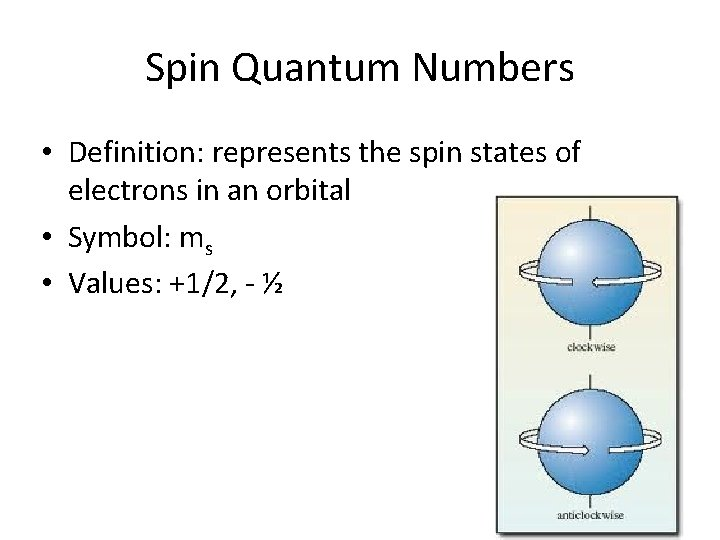Spin Quantum Numbers • Definition: represents the spin states of electrons in an orbital • Symbol: ms • Values: +1/2, - ½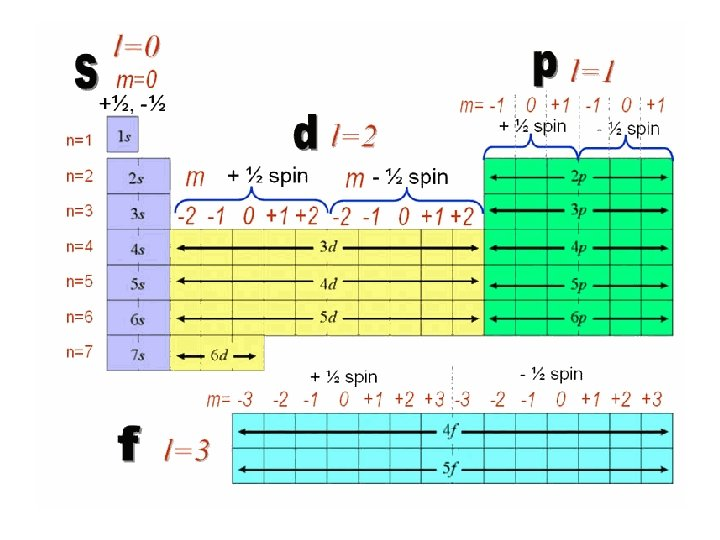Can an e- be described by the following set of quantum numbers? n=2, l=1, ml= -1 All quantum numbers are allowed values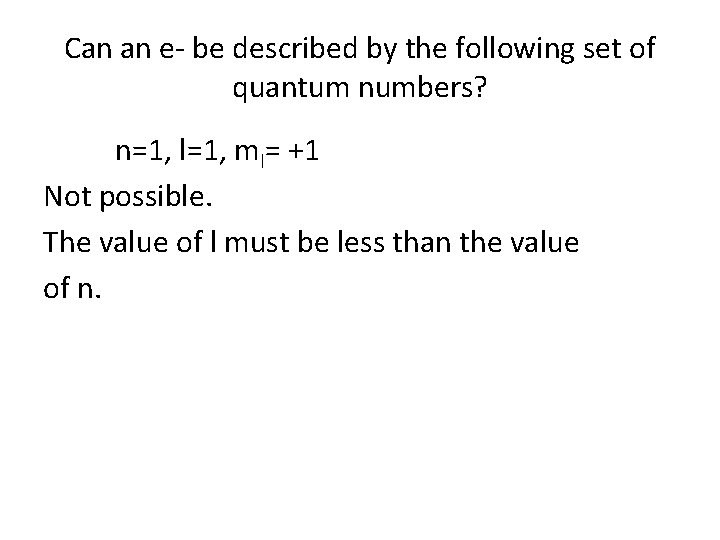Can an e- be described by the following set of quantum numbers? n=1, l=1, ml= +1 Not possible. The value of l must be less than the value of n.Can an e- be described by the following set of quantum numbers? n=7, l=3, ml= +3 All the quantum numbers are allowed values.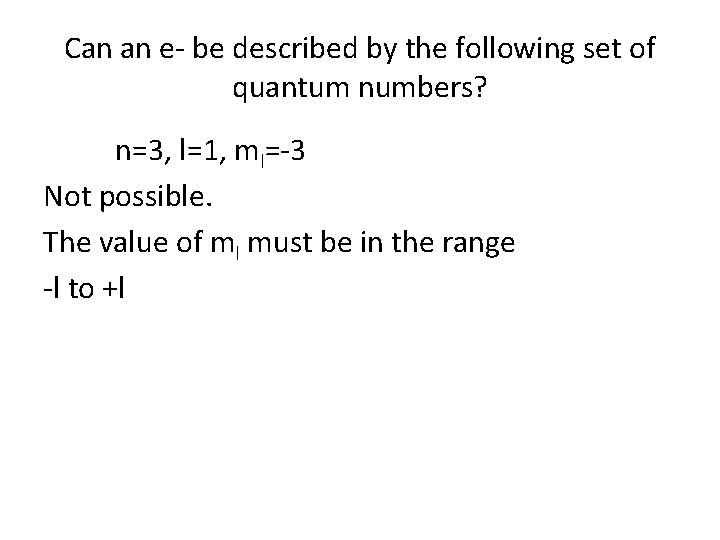Can an e- be described by the following set of quantum numbers? n=3, l=1, ml=-3 Not possible. The value of ml must be in the range -l to +l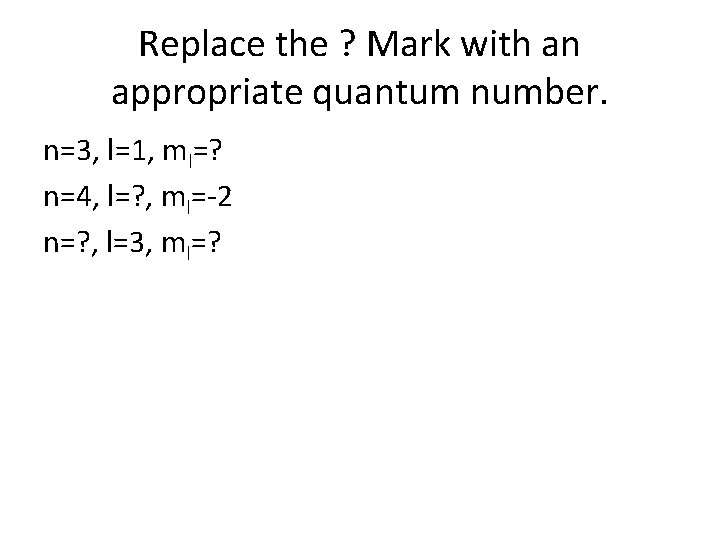Replace the ? Mark with an appropriate quantum number. n=3, l=1, ml=? n=4, l=? , ml=-2 n=? , l=3, ml=?Can an e- be described by the following set of quantum numbers? n=2, l=1, ml=-2 n=3, l=2, ml=+2 n=4, l=3, ml=+3 n=5, l=2, ml=+3Writing Quantum Numbers • You can identify the quantum numbers for any electron in an element • Start by writing out the electron configuration and orbital diagram for the element • Locate the electron in the orbital diagram that you are solving the quantum number for • Determine the 4 quantum numbersHydrogen’s electron • • • Write electron configuration Draw orbital diagram n= 1 l= 0 ms= +1/2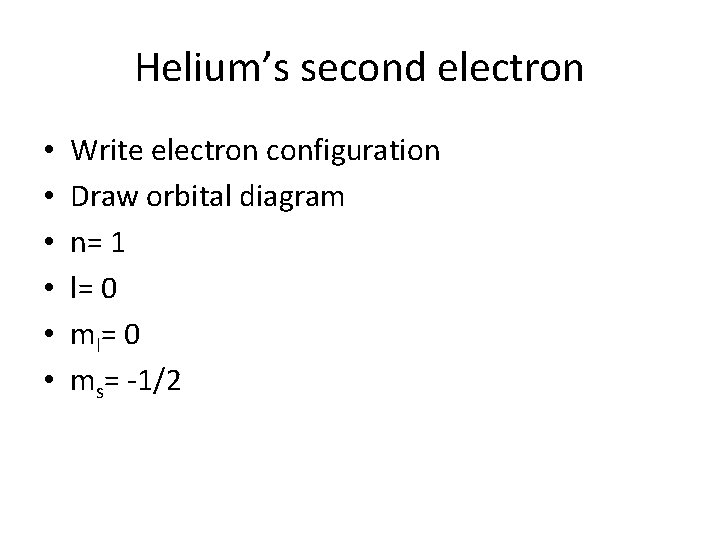Helium’s second electron • • • Write electron configuration Draw orbital diagram n= 1 l= 0 ms= -1/2Lithium’s third electron • • • Write electron configuration Draw orbital diagram n= 2 l= 0 ms= +1/2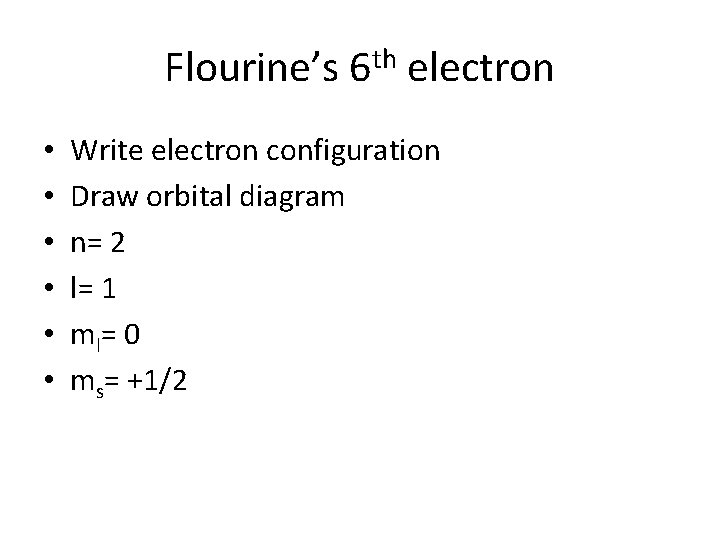Flourine’s 6 th electron • • • Write electron configuration Draw orbital diagram n= 2 l= 1 m l= 0 ms= +1/2